# Visualizing Early Addition and Subtraction Question Structures

In this Math Is Visual video animation and visual prompts, we will explore early addition and subtraction question structures through concrete and visual representations! Learn about joining, separating, part-part whole relationships and comparing relationships.

Early Addition and Subtraction is much more than memorizing key words and phrases like many of us tend to do to with the hope of students becoming fluent with an idea over time.

As students work through early addition and subtraction, they should be exposed to different types of addition and subtraction question structures including joining, separating, part whole or “parts” whole and comparing.

Engage in the following series of Math Is Visual Prompts and the instructional guide to promote fluency with addition and subtraction using visual representations rather than defaulting to key words or other nemonic devices that may disguise memorization as fluency.

Please note the addition and subtraction structures were based on the work of Van De Walle in Elementary and Middle School Mathematics: Teaching Developmentally and the questions were based on Marian Small’s work in Leaps and Bounds.

## Visual Prompt #1: Joining Addition Structure

3 chicks are eating.

4 more chicks come to eat.

How many chicks are there altogether?

At this point in the video pause or simply show the following image: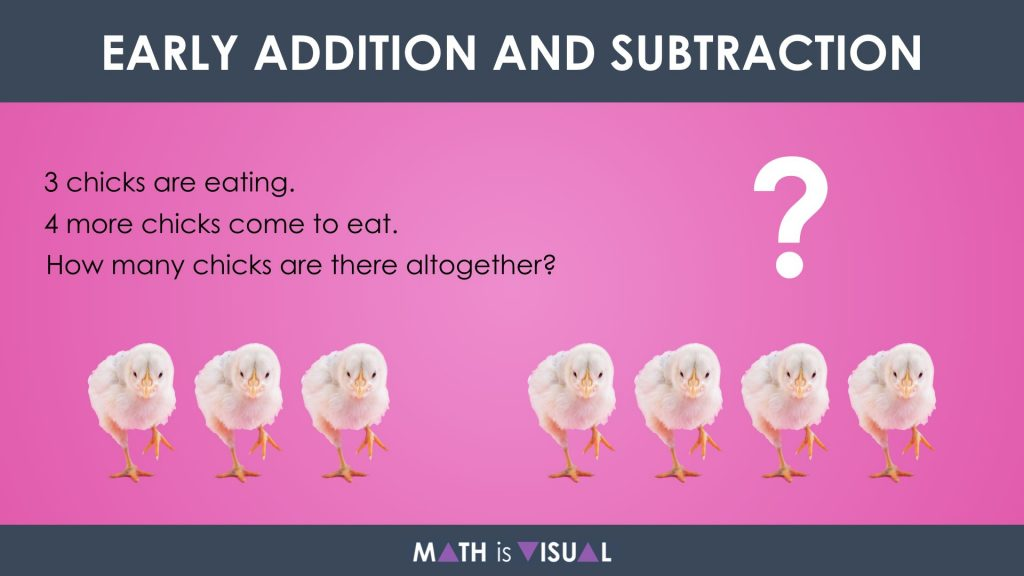Ensuring students have manipulatives like square tiles, linking cubes, counters, etc. on the table, allow them to use the manipulatives to help them answer this question.

In this particular problem, we can look at the structure of this addition problem as a joining problem where we have a:

• start;
• change; and,
• result.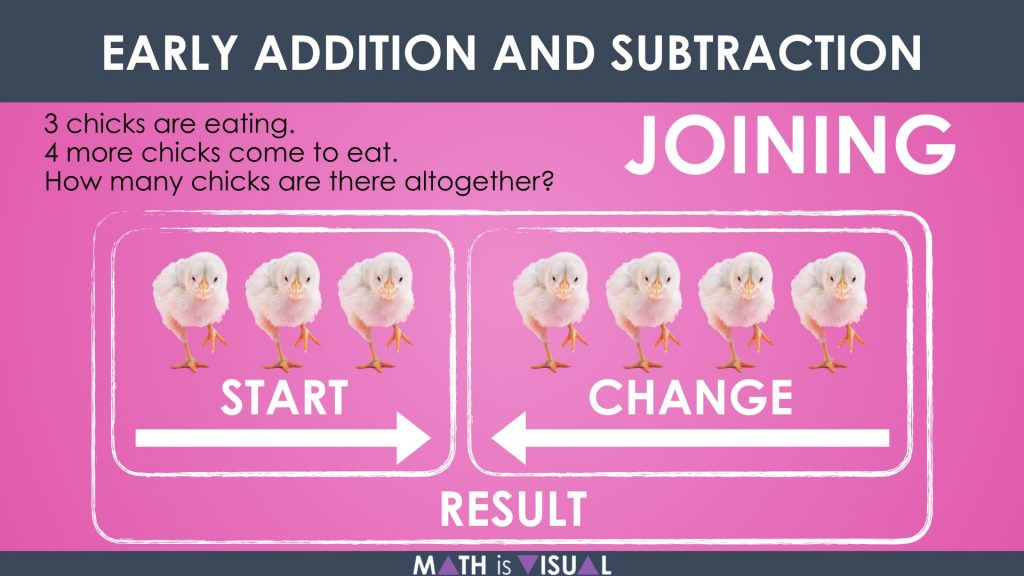Notice how the visual allows us to see the start and the change as the two “parts” (addends) of this problem.

## Visual Prompt #2: Separating Subtraction Structure

Here’s the second problem in the video:

There are 16 pigeons.

7 fly away.

How many are left?

Pause the video or show the following image to allow students to use their manipulatives to represent a solution.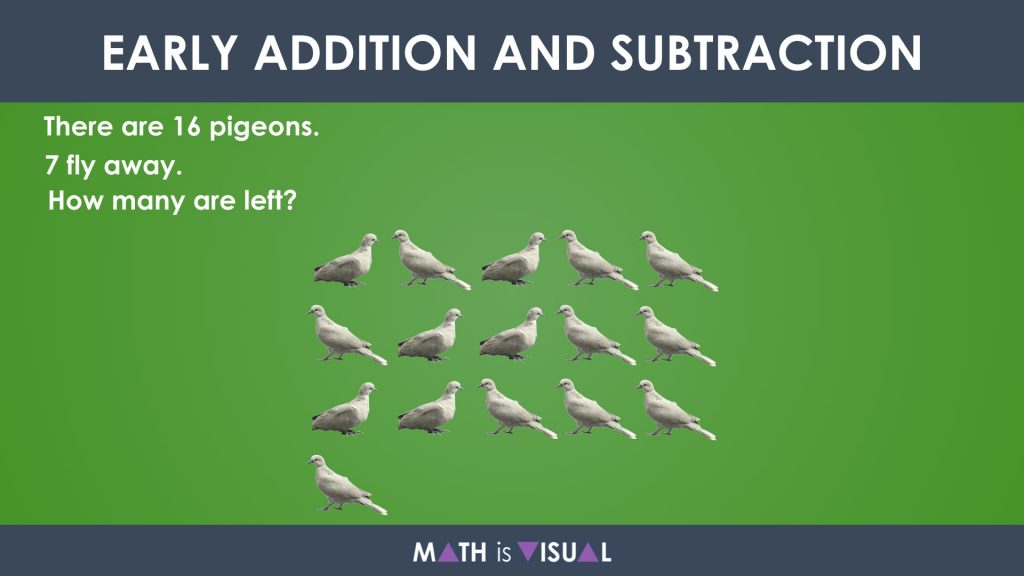Often times, we might feel it is helpful to put the word “left” on a wall or anchor chart of subtraction words. However, what can be much more powerful than this is to have students understand the full context of this problem involving the:

• start;
• change; and,
• result.

So rather than looking at subtraction as something completely unrelated to addition, we can leverage the intrinsic relationship that exists between addition and subtraction by leveraging very similar looking concrete and visual representations: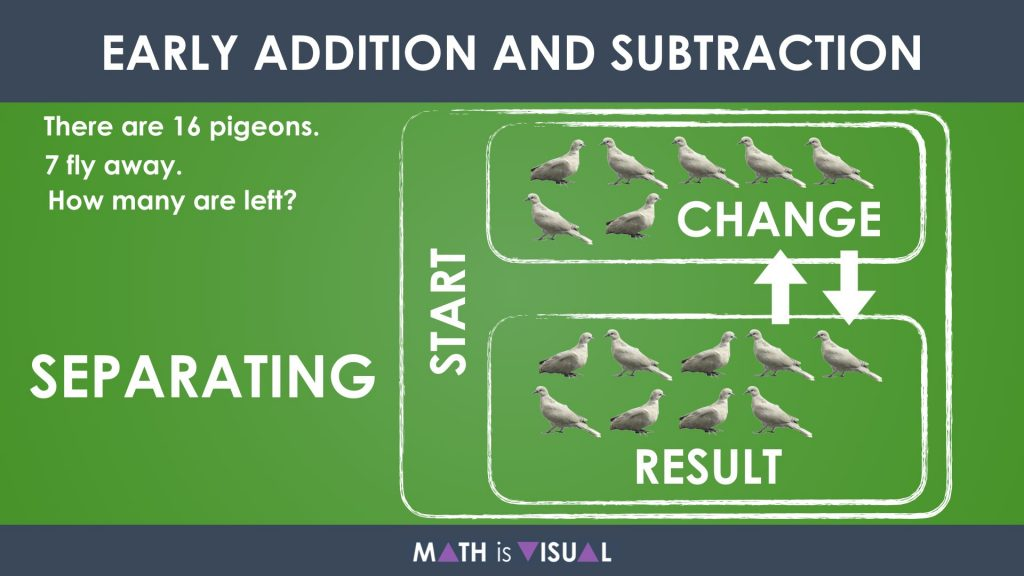Notice that this representation looks almost identical to that of the previous addition problem in the first visual prompt, however the start is the large set and the result is the smaller set. Since addition and subtraction are opposite operations, it would make sense that the opposite of the joining question structure would be called separating.

## Visual Prompt #3: Part-Part Whole Addition Structure

Pause the video and let the viewer apply their knowledge of positive number divided by a negative number using partitive division in the following visual prompt:

Raylah has 4 loonies.

Cole has 4 loonies.

How many loonies do they have in all?

Pause the video or show the following image: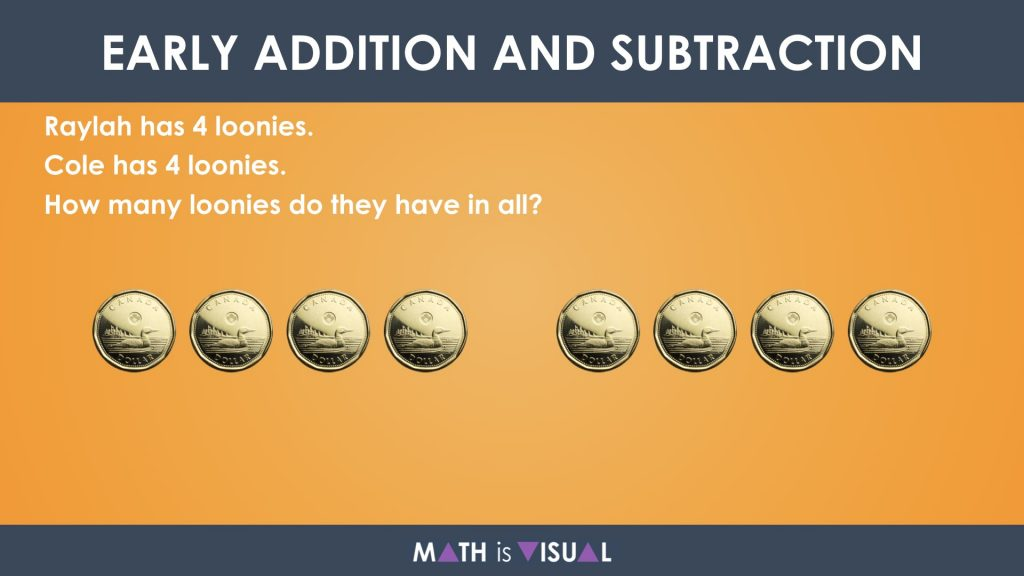When students attempt solving this problem, they can approach it using the joining addition structure since there is a start, change and result just like the previous two prompts:However, a 3rd structure or way of representing this problem can be through a part-part whole representation where we simply rename the start and change to “parts” and the result as the “whole“:Allow time for the viewer to engage in the partitive division before showing the remainder of the video animation or the following still image.

## Visual Prompt #4: Part-Part Whole Subtraction Structure

Just as the joining structure for addition can be reorganized as the separating structure for subtraction, the part-part whole structure for addition can also be repurposed for subtraction.

Let’s change the previous prompt just slightly:

Raylah has 8 loonies.

She gives Cole 4 loonies.

How many loonies does Raylah have left?

Pause the video or show the following image: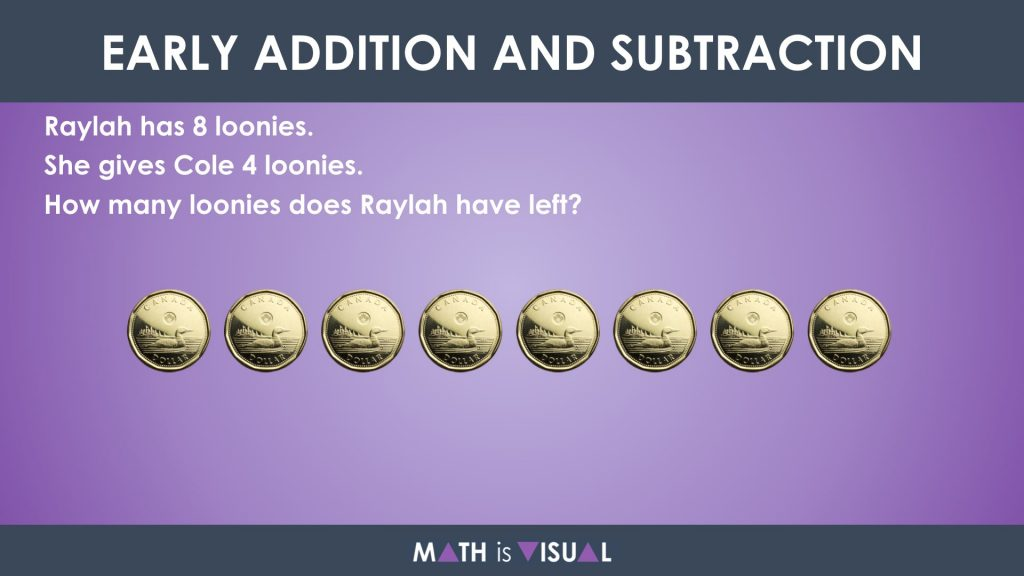As mentioned, we could use the separating subtraction structure since we were given a large set to start, we were given a change, and what we were left with is the result: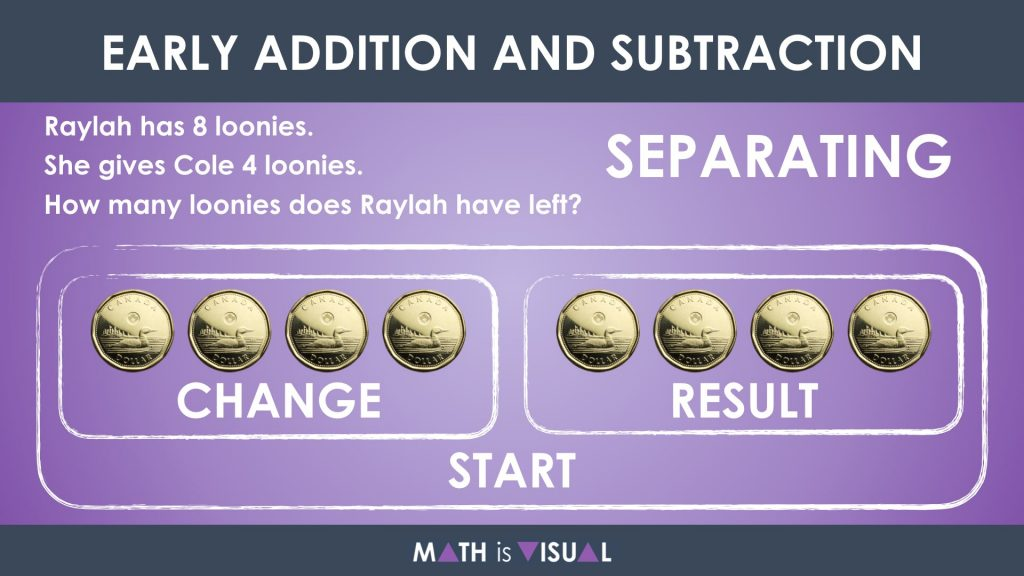We can just as easily (maybe more easily?) view this particular problem using the part-part whole structure for subtraction: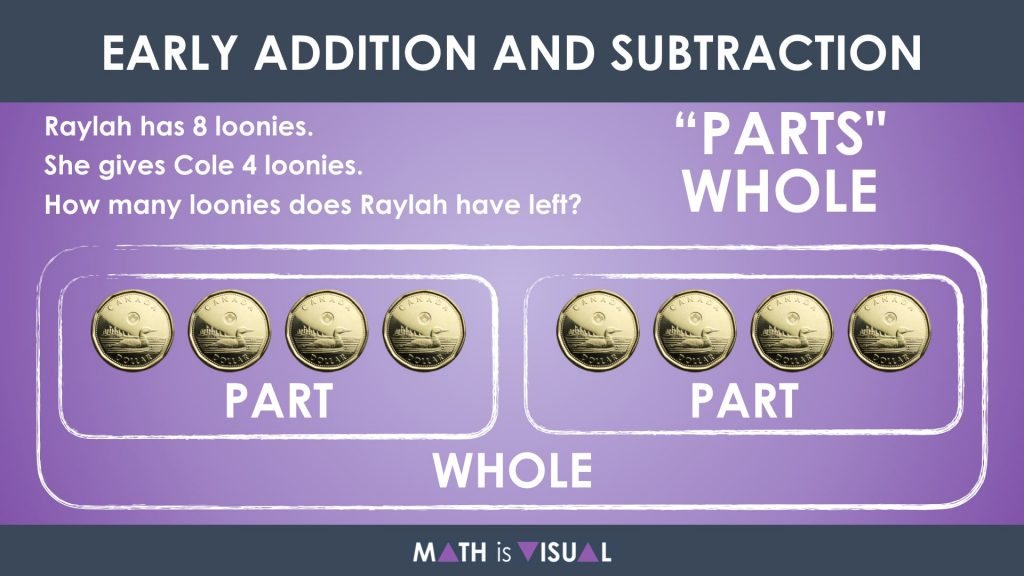Here, we were given the whole (8 loonies) and one of the parts (4 loonies). That left us attempting to determine the missing part (4 loonies).

## Visual Prompt #5: Comparison Subtraction Structure

The last structure we will look at in this series of Math Is Visual Prompts is the comparison subtraction structure.

Here’s the final question:

There are 5 bees.

You need 10 bees.

How many more bees do you need?

Pause the video or show the following image: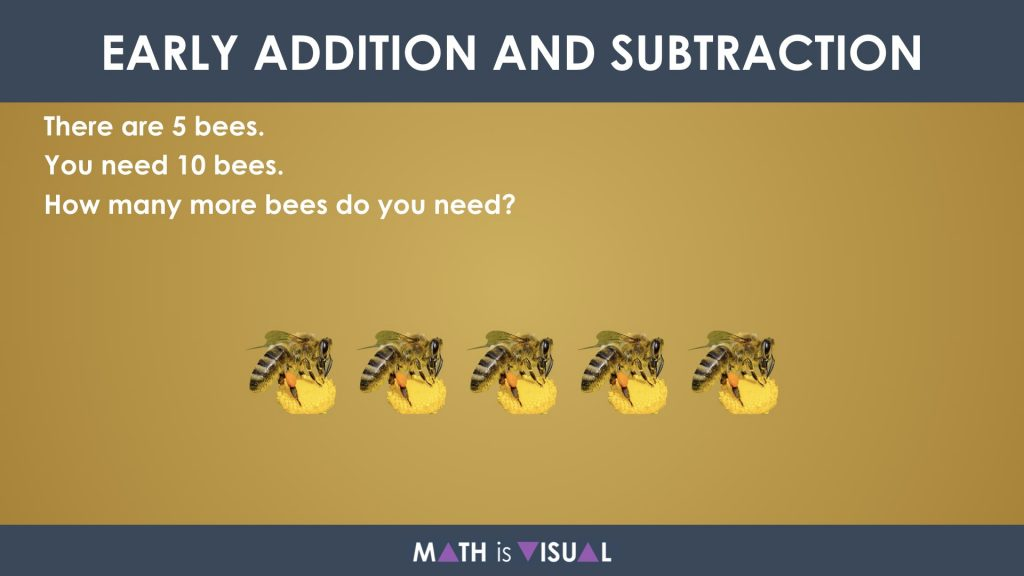Notice how in this problem, the word more is used and it is done so intentionally here to determine which students have a deep understanding of the problem and the mathematics they are being asked to complete and which students are memorizing key words. For example, the word more is often put on the “addition” list because it is often used to ask students how many more objects are in a set when adding.

In this case, we are subtracting.

Here is what this could look like when we consider this comparison subtraction structure problem: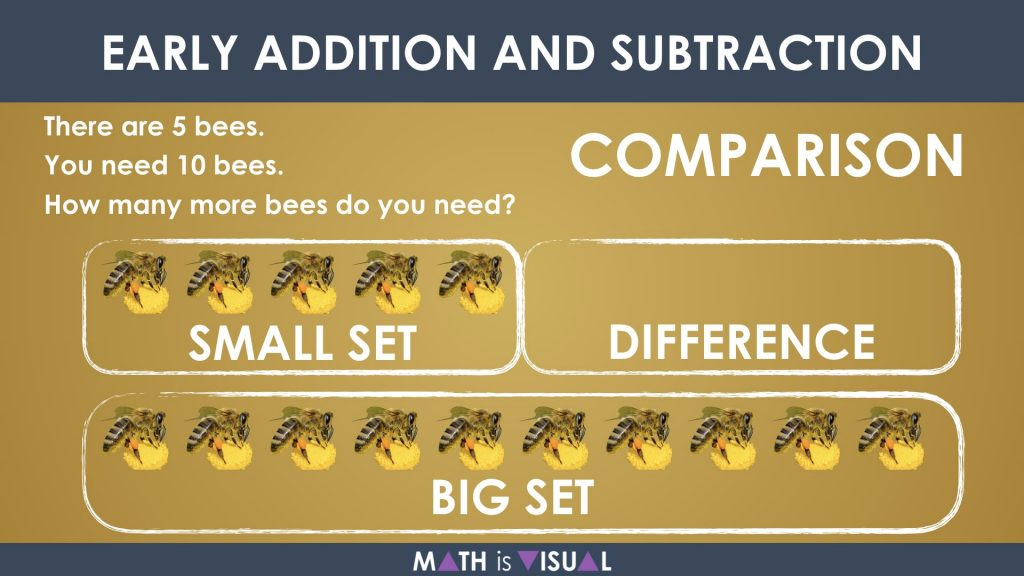It is important to note that we can also approach this problem using the part-part whole structure and it looks almost identical: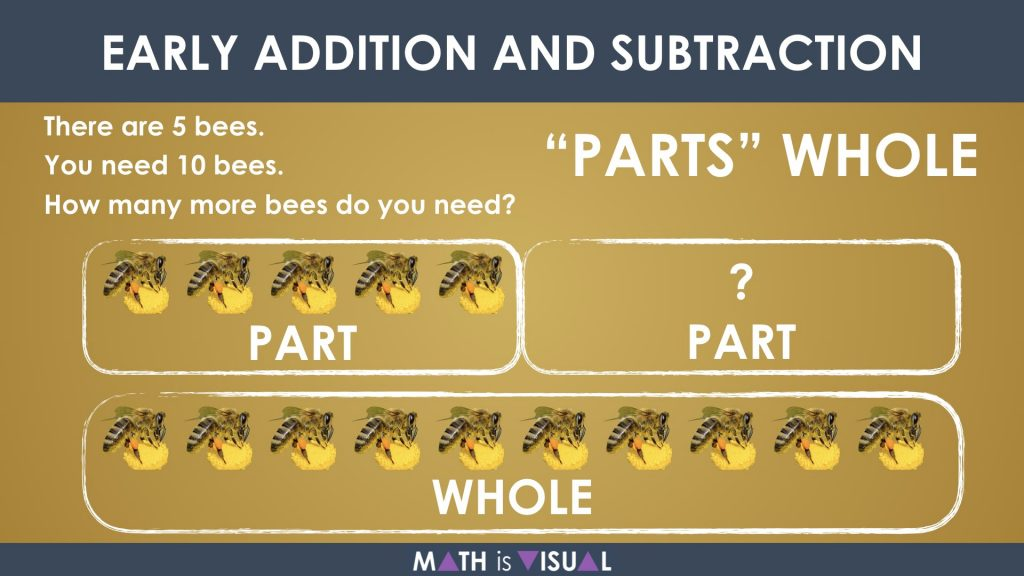## Four Structures That All Resemble Part-Part Whole

If you’re like me, when I analyze these addition and subtraction structures further, you can clearly see the part-part whole represented in each of the structures above:It’s no wonder that many math leaders like Ann Pigeon and many others often stress the importance of the part-part whole relationship. Not only can it be used to think about all four addition and subtraction question structures, but it also helps us to think algebraically and can be extended easily for solving equations.

Did you use this in your classroom or at home? How’d it go? Post in the comments!

Math IS Visual. Let’s teach it that way.

Dive into real world math lessons that engage students and also develop conceptual understanding and procedural fluency throughout.#### kylepearce3

•John Butler says:

Beautifully simple and visual representation .. as it should be.

• […] pretty complex ideas including subitizing, abstraction, and unitizing which quickly develops into addition, subtraction, multiplication and division. Then, possibly without even knowing it, kids find themselves in a […]

• […] student thinking forward in the area of addition and subtraction structures. Here are some other addition and subtraction structures you might consider checking out on my site Math Is […]

•Jeff says:

I’ve been looking for something like this for years! It’s such a clear and precise illustration of CGI/SBI word problem types. Thanks so much!

•kylepearce3 says:

So glad to hear it! Hope you enjoy!

•David Bugbillah says:

the illustrations used are simple and clear. I might say it makes learning of math easy and enjoyable. Will you permit me to use these illustrations in the my book that i will be publishing soon?

•kylepearce3 says:

Hi David,
Of course! If you could reference the website in your book, that would be fantastic.
Would love to give it a read once you’re ready to publish, too!